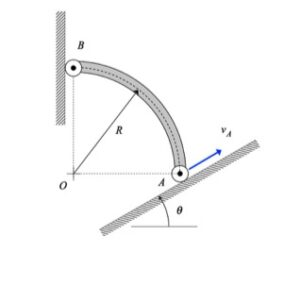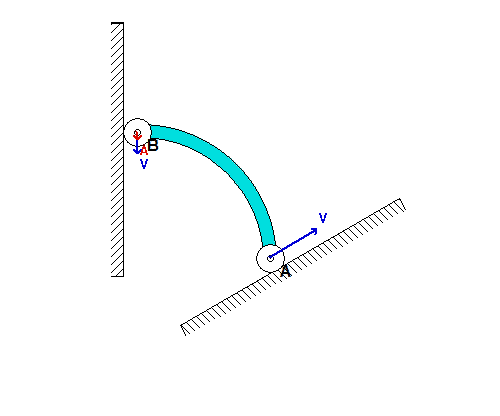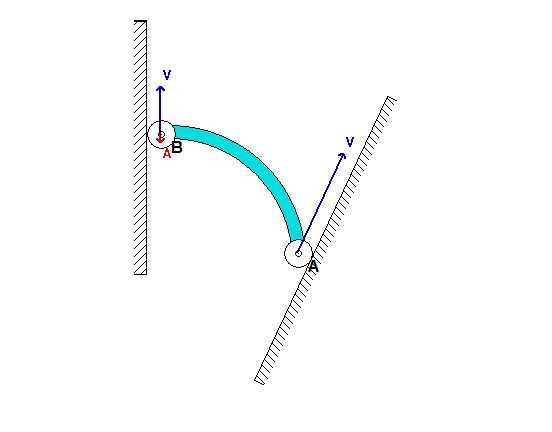# Homework H2.B - Sp23

 Problem statement Solution videoDiscussion and hints:

The solution for the velocity and acceleration of end B is a straight-forward application of the rigid body velocity and acceleration equations for member AB:

vB = vA + omega x rB/A
aB = aA + α x rB/A - ω2*rB/A

where vB = vB*j, vA = vA*(cos(θ)*i + sin(θ)*j), aB = aB*and aA = aA*(cos(θ)*+ sin(θ)*j)Each of the two vector equations above represents two scalar equations, providing us with the necessary equations to solve for vB, ω, aB and α.

HINT: The solution for this problem follows very closely that of Example 2.A.7 of the lecture book. If you get stuck on this problem, it is recommended that you review the solution video for Example 2.A.7 on the course website.For the inclination angle used in the above simulation, we see that point B moves DOWNWARD along the vertical wall as A moves up along the incline. As B moves onto the same horizontal plane as A, the acceleration of B becomes very large (although A continues to move with a constant speed). Can you provide a physical explanation for this?If we now consider a steeper inclination angle for A, as used above, we see that end B initially moves UPWARD along the wall; however, at some point B reverses its direction and begins to move DOWNWARD along the wall. Can you provide a physical explanation for this? Note also that the acceleration of B becomes very large as B moves onto the same horizontal plane as A, as it was for the initial value of inclination angle.

What is the value of the incline angle theta that defines the boundary between the types of initial motions for bar AB shown in the above two simulations? For the numerical value of the angle theta provided in the problem statement, which of the two simulations above agree with your results?

## 33 thoughts on “Homework H2.B - Sp23”

1.Sharon Ni says:

Can you assume that the acceleration of A is zero because the speed of vA is constant, for the acceleration vector for B?

1.Won Jun Lee says:

Yes, you can assume that the acceleration A is zero. Since the end of A's velocity is defined by a constant speed, the respective acceleration will be zero.

1.CMK says:

Picky point, but this is not an "assumption"; instead, it is a consequence of given information. Since A moves with a constant speed along a straight path, A has zero acceleration. No need to assume.

2.Myles Christian Kappes says:

The submission on gradescope is not appearing for me. Is this happening to anyone else?

1.Qianyu Zhao says:

It should be there now. Let me know if you still have that issue.

3.J Cena says:

I have both a and v in terms of w, but I am struggling to get w in terms of v and r.

1.. says:

I separated into i and j components similar to example 2.A.7 then solved for w in terms of v and r

2.Qianyu Zhao says:

Recall the end B of the bar is constrained to move in y only, then can it have any velocity and acceleration in x? This problem is also similar to HW 2.A part b in terms of approach, so you may find some comments there helpful.

4.Lily Waterman says:

I cannot figure out how to find angular velocity, but I know it should be in the k direction. Isn't angular velocity and velocity related somehow through R? Additionally, since the acceleration of A is 0, would the angular acceleration be 0 as well?

1.Matthew Issac Boxberger says:

Angular velocity is calculated by taking the velocity tangent to the radius and dividing it by the radius value.

1.Rongkai Yu says:

I don't think that's right for this question. I found the angular velocity using the velocity equation. Since there's no velocity in the x direction for B, any components that affect the i component of B would cancel out, and this gave me the angular velocity in end.

1.Nadra Elon Dunston says:

But what are we supposed to find v_B relative to? The coordinate origin?

1.Zachary William Delahunty says:

I believe we are supposed to find v_B relative to A since the equation is finding the velocity of B as observed from A

1.CMK says:

v_B is not relative to A.

In the equation, v_B = v_A + omega x r_B/A, v_B and v_A are the velocities of A and B as seen by a stationary observer.

omega x r_B/A is the velocity of B relative to A, as stated in the lecture book.

5.Sam Plumer says:

Just to make sure, when calculating the position vector rb/a it should be the vector pointing from a to b correct? I feel that I did everything correct but my answers for velocity and acceleration came out in the positive j direction, which does not make sense.

1.Branson Dao says:

Yes, basically r_b/a is the position vector b with respect to a (I believe that is how it's said); this means that B is the final position and A is the starting position.

6.Madeline B says:

I just want to confirm that I am on the right path. Is the rB/A = R or are we doing the arc length equation again? So rB/A = pi*R / 4?

1.Gage Alan Wilson Ernsberger says:

I didn't use the arc length formula. It can be seen since 0 is directly below B and to the left of A. I did R_b = Rj and R_a=Ri. From here it can be seen that Rb/a = R_b-R_a. This gives all our equations to be in i and j

1.Ella says:

Can we use this since it is no longer Rbi-Raj once it starts moving? In the example in class the vector was a function of the angle between Ra/b and the horizontal, but I'm not sure how we would get that angle in this problem.

7.Michael Bradley Mayhew says:

When finding the position vector, do we need to use the arc length multiplied by cos/sin of the angle, or can we just use R * cos/sin?

8.Jacob Russell Bunton says:

Since omega = theta_dot and alpha= theta_ddot and theta is constant would that mean that omega and alpha would be zero?

9.Trisha Boodhoo says:

I looked at the example video 2.a.7 and I am confused - if a point is moving only in the j direction why does it follow that acceleration is in terms of j only? Does acceleration have to be parallel to the path of the point?

1.CMK says:

Trisha,

Think back to the second day of class. Then, we said that acceleration has two orthogonal components: one tangent to the path and one normal to the path. Here, "j" is tangent to the path, and "i" is normal to the path. The path is straight (rho = infinity), so the normal component of acceleration is zero. All that remains is the tangential component, and that is in the "j" direction. Hence, the acceleration must be in only the "j" direction.

Does this help?

10.Nadra Elon Dunston says:

I'm supposing omega and alpha are meant to be found using the velocity and acceleration of B. But what are we supposed to find v_B and a_B relative to? The origin or point A?

1.Nadra Dunston says:

Or it may be the other way around - find omega via A and use that to find v_B? Still, what would v_A be relative to?

2.CMK says:

You are asked to find the absolute velocity and acceleration, not relative to anything that moves.

1.Nadra Dunston says:

But the equation for velocity is vB = vA + omega x rB/A. The position vector rB/A is vecotr rB relative to a point A. So, when I find the velocities in this problem, are the position vectors relative to the opposite ends of the rigid body or the still origin O? Does 'absolute' velocity & acceleration always mean relative to the coordinate origin?

1.CMK says:

In that equation, v_B and v_A are absolute velocities (velocities seen from a stationary observer). In that equation, however, you need to use the position of B measured from the position of A, r_B/A.

The term omega x rB/A is the velocity of B relative to A.

11.Phillip Eugene Holmes says:

Do we need to use the arc length? It would seem that we just need to multiply R by cos and sin to get the i and j components. Then once we get these components we plug them into the equation Vb bar = Va bar + omega x rb/a. From here break up the equation and solve for the i component since we know its zero. This would get us the omega value which we can then use to find j. Is this the correct thought process with this problem?

1.CMK says:

Phillip: You need only the position vector from A to B: r_B/A = -R*i + R*j. No arc length is needed, nor does the angle theta come into play on that position vector.

1.Phillip Eugene Holmes says:

since we are finding absolute velocity and acceleration would both be positive values?

1.CMK says:

"Absolute" here = not "relative". It does not mean absolute value.

2.Alex L says:

Nah, you don't need to use arc length. You pretty much just draw a line from point A to point B and then you'll realize that you have a right triangle. And then from there you just do the normal trig stuff to get the i and j components for r_B/A.